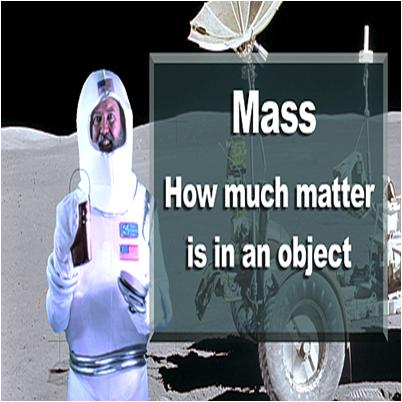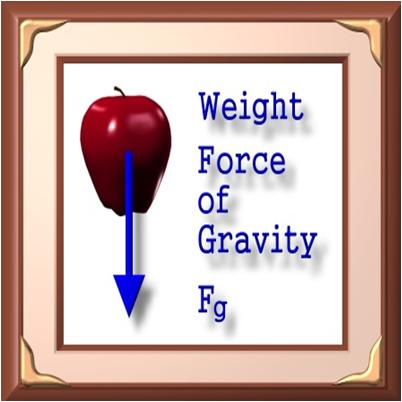# Difference Between Mass and Weight

Mass and weight are often confused with each other. They are frequently used as synonyms in regular language and discourse. The science students and even scientists sometimes find it difficult to differentiate and use them interchangeably.  On the other hand, mass and weight are quite different from each other, possessing many distinct qualities. Once you thoroughly understand the differences between mass and weight, you would be able to use them properly and according to the requirement.

Mass is the quantity of matter present in a matter (object) whereas Weight is a force with which the earth pulls the matters towards its center.

Mass is a scalar quantity – that has magnitude only and is not related to direction. Weight, on the other hand, is a vector quantity – that possesses both direction and magnitude.

Mass is an “intrinsic property” of an object and, therefore, remains stable (constant) at any place and time in the universe.  But weight keeps on changing with the change in the magnitude of the gravitational force.

Mass of an existing object can never be zero while Weight can be zero if there is no gravitational force acting on the object, like that in the space.

Mass is indestructible (imperishable) while weight can be decreased with less gravitational force and can be increased with more gravitational force.

### Instructions

• 1

Mass

“The quantity of matter possessed by the substances is called as Mass.”  It is an independent quantity and remains stable in every part of the world. Kilogram (kg), Grams (g), and Milligram (mg) are the units used to measure mass. The formula for mass is, m = f/a. “f” is the force of the object while “a” is the acceleration of the object (gravitational force).

Image Courtesy: thehappyscientist.com• 2

Weight

“A measurement of the gravitational force acting on an object is called as Weight.” It depends upon the value of the gravitational force and is expressed in Newton (N). Weight of an object fluctuates with the changes in the gravitational force, acting on it. A spring balance is used to calculate weigh of the matters.  The formula to compute is, Weight = mass x acceleration due to gravity.

Image Courtesy: zonalandeducation.com•